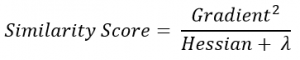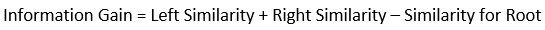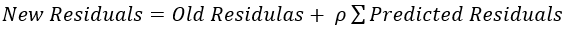Boosting

Classification

Decision Tree

Ensemble Technique

Machine Learning

Regression

January 15, 2021

# Explain the step by step implementation of XGBoost Algorithm.

XGBoost is an abbreviation of Extreme Gradient Boosted trees. Now you may ask, what is so extreme about them? and the answer is the level op optimization. This algorithm trains in a similar manner as GBT trains, except that it introduces a new method for constructing trees.

Trees in other ensemble algorithm are created in the conventional manner i.e. either using Gini Impurity or Entropy. But XGBoost introduces a new metric called similarity score for node selection and splitting.

Following are the steps involved in creating a Decision Tree using similarity score:

1. Create a single leaf tree.
2. For the first tree, compute the average of target variable as prediction and calculate the residuals using the desired loss function. For subsequent trees the residuals come from prediction made by previous tree.
3. Calculate the similarity score using the following formula:where, Hessian is equal to number of residuals; Gradient2 = squared sum of residuals;  λ is a regularization hyperparameter.
4. Using similarity score we select the appropriate node. Higher the similarity score more the homogeneity.
5. Using similarity score we calculate Information gain. Information gain gives the difference between old similarity and new similarity and thus tells how much homogeneity is achieved by splitting the node at a given point. It is calculated using the following formula:6. Create the tree of desired length using the above method. Pruning and regularization would be done by playing with the regularization hyperparameter.
7. Predict the  residual values using the Decision Tree you constructed.
8. The new set of residuals is calculated using the following formula:where ρ is the learning rate.
9. Go back to step 1 and repeat the process for all the trees.

by : Monis Khan

Quick Summary:

XGBoost is an abbreviation of Extreme Gradient Boosted trees. Now you may ask, what is so extreme about them? and the answer is the level op optimization. This algorithm trains in a similar manner as GBT trains, except that it introduces a new method for constructing trees. Trees in other ensemble algorithm are created in […]